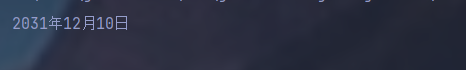# JavaSE系列基礎包裝類及日歷類詳解

## 1. 基本類型包裝類

### 1.1 概述```public class IntegerDemo {
public static void main(String[] args) {
//判斷數據是否在int范圍內
System.out.println(Integer.MAX_VALUE);//最大范圍
System.out.println(Integer.MIN_VALUE);//最小范圍
}
}
```

### 1.2 Integer類的概述與使用```Integer i3=new Integer("asd");//這裡就會運行錯誤
```

```public class IntegerDemo {
public static void main(String[] args) {
Integer i1=Integer.valueOf(123);//數字類型
System.out.println(i1);
Integer i2=Integer.valueOf("123");//字符串類型
}
}

```

### 1.3 int和String的相互轉換

```int number=100;
String s1=""+number;
```String—>Integer—>int

```public class IntegerDemo {
public static void main(String[] args) {
int num=100;
String s1=String.valueOf(num);
//int轉換為字符串類型
System.out.println(s1);
System.out.println("----------");
//字符串轉換為int
String s2="100";
// 方式一:
//String--->Integer--->int
Integer i1=Integer.valueOf(s2);
//通過幫助文檔發現，
// intValue()用來返回Integer
// 作為int的值，正好符合要求
int x=i1.intValue();//返回值用int接收
System.out.println(x);
//方式二：使用parseInt靜態方法
int y=Integer.parseInt(s2);
System.out.println(y);
}
}
```

### 1.4 案例-字符串中數據排序```public class IntegerDemo {
public static void main(String[] args) {
//定義字符串
String s="91 27 46 38 56";
//將字符串中數據抽離出來,以空格作為分割點
String[] s1 = s.split(" ");
//定義一個int數組，用於存這些數據
int []arr=new int[s1.length];//以s1的長度
//轉換為int類型
for (int i = 0; i < s1.length; i++) {
arr[i] = Integer.parseInt(s1[i]);
//賦值
}
//進行排序
Arrays.sort(arr);
//字符串拼拼接
StringBuilder sb=new StringBuilder();
for(int i = 0; i < arr.length; i++){
if(i==arr.length-1){
sb.append(arr[i]);
}else{
sb.append(arr[i]).append(" ");
}

}
String result = sb.toString();
System.out.println("排序後的："+ result);

}
}
```

### 1.5 自動裝箱和拆箱

```Inter i=Integer.valueOf(100);//這裡先把100轉化為Inter類型，賦值
Integer i1=100;//這裡java5之後就在jdk內部
//已經潛移默化的為我們包裝好瞭
//它等同於上步
```

```Integer i1=100;
//拆箱：轉換為int型，用intValue()這個方法
//然後進行基本類型的運算後再賦值給i1
//完成自動拆箱
i1=i1.intValue()+200;//最後賦值是自動裝箱
```

```Integer i1=100;
i1+=200;//內部隱含瞭自動拆箱和自動裝箱
```

## 2. Date類Date 代表瞭指定時間，精確到毫秒```public class DateDemo {
public static void main(String[] args) {
Date d=new Date();
//按道理輸出的應該是地址，結果輸出瞭時間，因為重寫瞭toString方法
System.out.println(d);
//有參構造
long data=1000*60*60;//毫秒*1000->秒->*60->分鐘->*60->小時
Date d2=new Date(data);
System.out.println(d2);
}
}
```

Wed Dec 15 17:51:04 CST 2021

Thu Jan 01 09:00:00 CST 1970//其中09:00:00是時區

### 2.1 Date常用方法

public long getTime() 獲取的是日期對象，從1970年1月1日00：00：00到現在的毫秒

public void setTime(long time) 設置時間，給的是毫秒值

```getTime:
public class DateDemo {
public static void main(String[] args) {
Date d=new Date();
//獲取時間
System.out.println(d.getTime()*1.0/1000/60/60/24/365+"年");
}
}

```

51.99024939979706年//從1970到現在

```setTime
public class DateDemo {
public static void main(String[] args) {
Date d=new Date();
long date=1000*60*60;
d.setTime(date);
System.out.println(d);
}
}
```

Thu Jan 01 09:00:00 CST 1970

## 3. SimpleDateFormat類

SimpleDateFormat是一個具體的類，用於以區域設置敏感的方式格式化和解析日期。重點學習日期格式化和解析化

• y…年
• M…月
• d…天
• H…時
• m…分
• s…秒SimpleDateFormat格式化與解析日期

```import java.text.SimpleDateFormat;
import java.util.Date;

public class SimpleDateFormatDemo {
public static void main(String[] args) {
//格式化
Date d=new Date();
//無參構造
//先創建SimpleDateFormat類
SimpleDateFormat sdf = new SimpleDateFormat();
//通過對象調用方法
String s = sdf.format(d);
System.out.println(s);

}
}
```

2021/12/15 下午7:11

``` SimpleDateFormat sdf = new SimpleDateFormat("yyyy年MM月dd日 HH:mm:ss");
```2021-12-15 12:00:00要和yyyy-MM-dd HH:mm:ss”格式對應，例如都需要-,在代碼下面會提示異常，要拋出異常，快捷鍵Alt+Enter

```import java.text.ParseException;
import java.text.SimpleDateFormat;
import java.util.Date;

public class SimpleDateFormatDemo {
public static void main(String[] args) throws ParseException {//拋出異常
//解析
Date d=new Date();
String s="2021-12-15 12:00:00";
//先創建SimpleDateFormat類
SimpleDateFormat sdf = new SimpleDateFormat("yyyy-MM-dd HH:mm:ss");//這裡格式要和模式的一樣
//通過對象調用方法
Date ss = sdf.parse(s);
//拋出異常Alt+Enter
System.out.println(ss);

}
}

```

### 3.1 案例-日期工具類```import java.util.Date;
import java.text.ParseException;
import java.text.SimpleDateFormat;

/*

*/
public class DateUtils {
private DateUtils() {}
//方法，把日期轉化為指定格式的字符串
//返回類型：String
//參數：Date date,String format
public static String dateTostring(Date date,String format){
SimpleDateFormat sdf=new SimpleDateFormat(format);
String s = sdf.format(date);
return s;
}
//方法，把字符串轉化為指定格式的日期
//返回類型：Date
//參數：String s,String format
public static Date stringTodate(String s1,String format) throws ParseException {
SimpleDateFormat sdf=new SimpleDateFormat(format);
Date p = sdf.parse(s1);
return p;
}
}
```

```import java.text.ParseException;
import java.text.SimpleDateFormat;
import java.util.Date;

public class SimpleDateFormatDemo {
public static void main(String[] args) throws ParseException {//拋出異常
//創建日期對象
Date d=new Date();
//調用方法
String s = DateUtils.dateTostring(d, "yyyy年MM月dd日 HH:mm:ss");
System.out.println(s);
//也可以拿到部分
String s1 = DateUtils.dateTostring(d, "yyyy年MM月dd日");
System.out.println(s1);
System.out.println("----以下是解析----");
String s2="2021-12-15 00:00:00";
Date date = DateUtils.stringTodate(s2, "yyyy-MM-dd HH:mm:ss");
System.out.println(date);
}
}
```## 4. Calendar類-日歷

Calendar為某一時刻和一組日歷字段之間的轉換提供瞭一些方法，並為操作日歷字段提供瞭一些方法

Calendar提供瞭一個類方法getlnstance用於獲取Calendar對象，其日歷字段已使用當前日期和時間```import java.util.Calendar;

public class CalendarDemo {
public static void main(String[] args) {
//獲取對象
Calendar c = Calendar.getInstance();//多態的形式
int year = c.get(Calendar.YEAR);//因為字段是靜態修飾的，所以通過對象調用字段
//即Calendar.YEAR
int month = c.get(Calendar.MONTH)+1;//一定要註意，月份是從0開始的，要+1
int date = c.get(Calendar.DATE);
System.out.println(year+"年"+month+"月"+date+"日");
}
}
```

2021年12月15日

### 4.1 Calendar 的常用方法```import java.util.Calendar;

public class CalendarDemo {
public static void main(String[] args) {
//獲取對象
Calendar c = Calendar.getInstance();//多態的形式
int year = c.get(Calendar.YEAR);//因為字段是靜態修飾的，所以通過對象調用字段
//即Calendar.YEAR
int month = c.get(Calendar.MONTH)+1;//一定要註意，月份是從0開始的，要+1
int date = c.get(Calendar.DATE);
System.out.println(year+"年"+month+"月"+date+"日");
}
}
```set()方法

```import java.util.Calendar;

public class CalendarDemo {
public static void main(String[] args) {
//獲取對象
Calendar c = Calendar.getInstance();//多態的形式
c.set(2022,11,05);//這裡11出來的結果會是12
int year = c.get(Calendar.YEAR);//因為字段是靜態修飾的，所以通過對象調用字段
//即Calendar.YEAR
int month = c.get(Calendar.MONTH)+1;//一定要註意，月份是從0開始的，要+1
int date = c.get(Calendar.DATE);
System.out.println(year+"年"+month+"月"+date+"日");
}
}
```